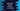# How to convert a string to an array of characters in TypeScript using split### Convert a string to array in TypeScript using split:

In this post, we will learn how to convert a string to an array in TypeScript. Basically the array will hold all the characters of the string. We can achieve this in different ways and I will explain these ways with examples in this post.

### String with comma separated characters:

For a string with comma separated characters, we can use split. split optionally can take one character and it splits the string where it finds that character. For example,

``````let given_str = 'a,b,c,d,e,f,g';

let final_arr = given_str.split(',');
console.log(final_arr);``````

If you run the above program, it will give the below output:

``[ 'a', 'b', 'c', 'd', 'e', 'f', 'g' ]``

Here,

• final_arr is the final array created by using split
• As you can see here, final_arr holds the characters of the string given_str

### Number string to array of digits:

Using split, we can convert a string of digits to an array of digits in the string. We need to use map to map each character to Number. Below is the complete program:

``````let given_str = '123456789';

let final_arr = given_str.split('').map(Number);
console.log(final_arr);``````

It will print the below output:

``[ 1, 2, 3, 4, 5, 6, 7, 8, 9 ]``

Here,

We are not passing any argument to the split function. So, it is splitting all numbers of the string.

### Things to keep in mind while using split:

split will consider one blank space as a character and if we have blank spaces in the string, it might give some unexpected results. For example:

``````let given_str = 'hello world';

let final_arr = given_str.split('');
console.log(final_arr);``````

It will give the below output:

``[ 'h', 'e', 'l', 'l', 'o', ' ', 'w', 'o', 'r', 'l', 'd' ]``

Here, you can see that it added the blank space to the string.

### Using with smileys:

split is not a right way to use with smileys. For example:

``````let given_str = 'hello😀world';

let final_arr = given_str.split('');
console.log(final_arr);``````

It will print:

``[ 'h', 'e', 'l', 'l', 'o', '�', '�', 'w', 'o', 'r', 'l', 'd' ]``

This is because, the smiley used is actually UTF-8 encoded. And, it is consturcted with two characters.